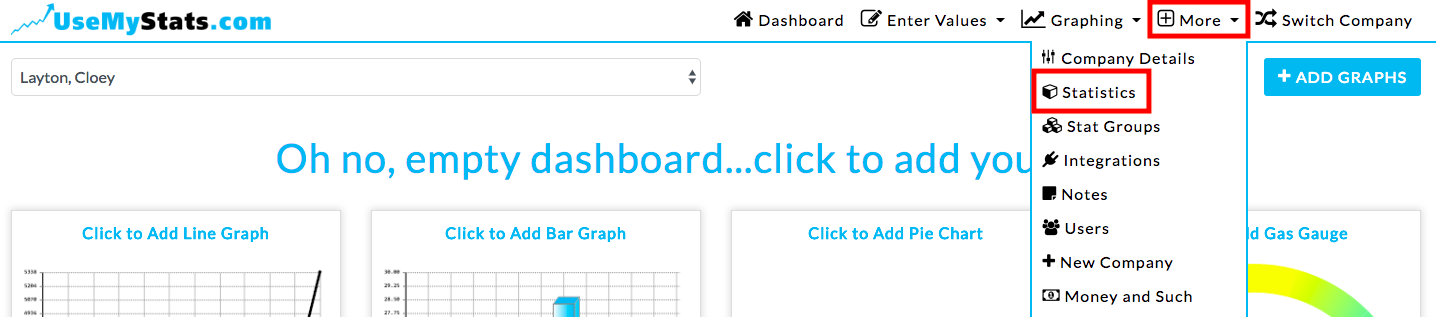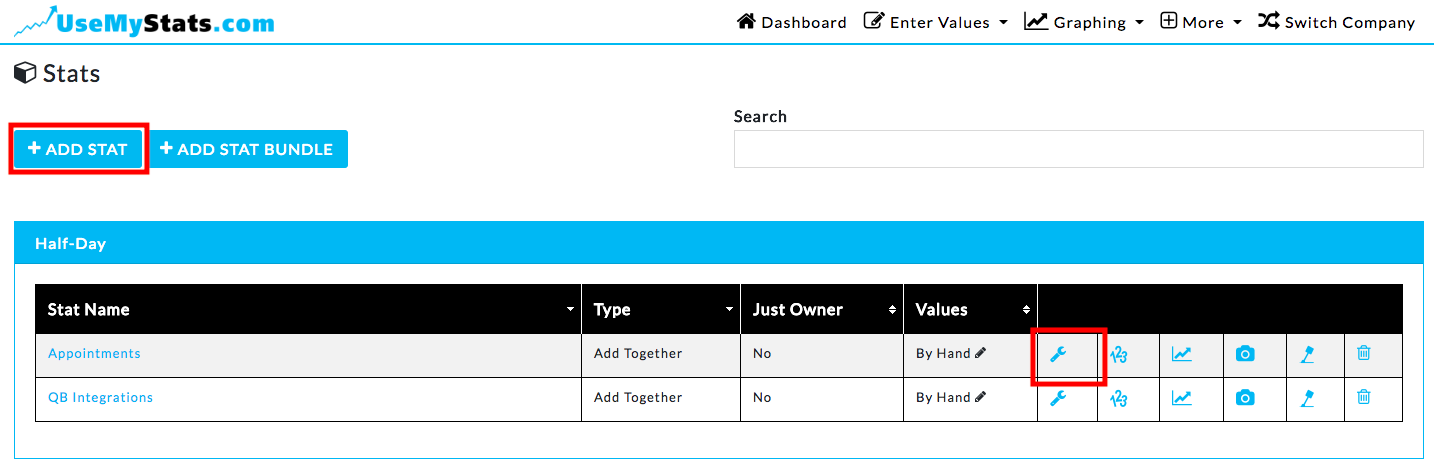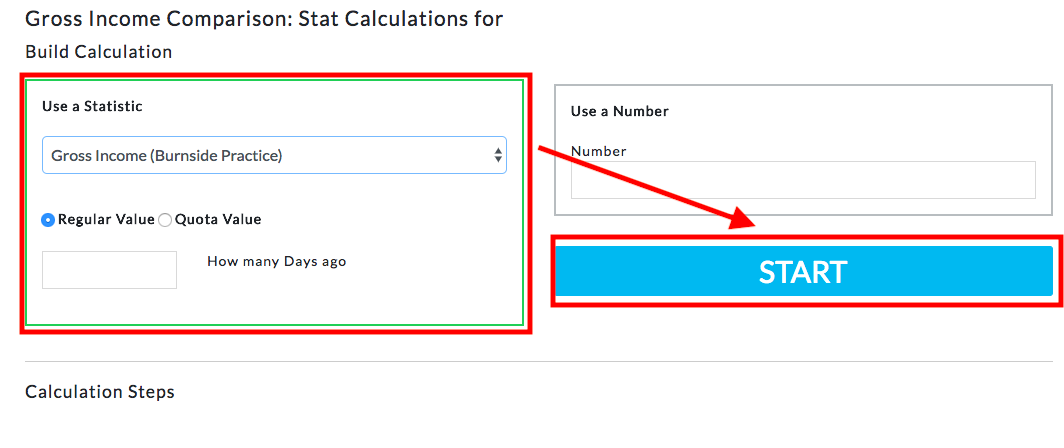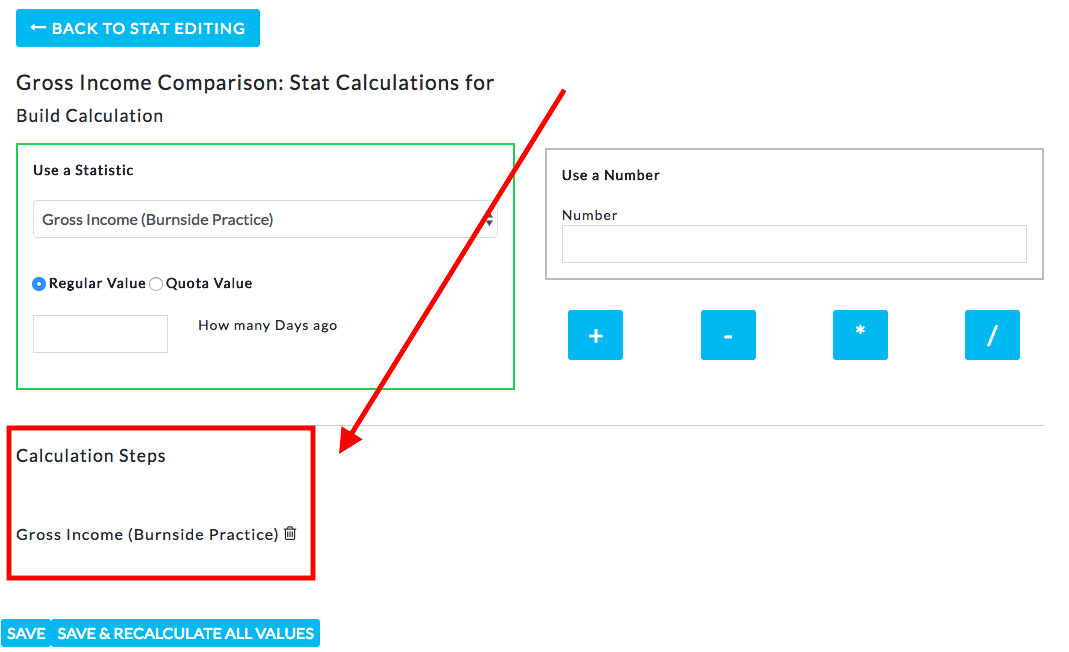Calculations are set up from the statistic editing page through “More”, then “Statistics”.Once you’ve clicked either “Add Stat” for a new one, or the wrench icon to edit an existing stat, the statistic settings page displays.Now click the arrow next to “Advanced Settings” to display the full settings options.Change the Values dropdown box to Calculation, and then click the “Set Up Data” button.The first thing to decide is whether you’re using an existing statistic or a number as the base of your calculation. In this case, I want to generate a Net Income statistic which will rely on the Gross Income and Gross Expenses statistics, so I’m beginning with the Gross Income statistic.

First select the statistic in the dropdown box, then select whether you’re using the actual values or quota values in your calculation, and finally whether you want to use the current values (leave the “how many weeks ago” box empty), or compare to previous time frames. The “How many weeks ago” box allows you to compare current values to earlier values for the same statistic. This is often used to generate percentages. Now click the “Start” button.The section below now shows the first step of your calculation, here beginning with Gross Income. To remove the step, just click the delete button.Now, select the second step using the same process. Either another stat (in this case I’m using Gross Income ), or by a number. For example, I could now do Gross Income / 2. Where the “Start” button was before, you now select the operator. In this case, after selecting Gross Expenses, I choose “-”. Again, the operation is added to the calculation below.Once you’re done building the calculation, you can use the “Save” button which will begin calculating on the next interval (the upcoming day, week, month, etc. based on how often this stat is reported), or use the “Save & Recalculate All Values” button which will go back in time to the earliest interval that values are entered for the statistics used in the calculation. For example, if I started entering the Gross Income and Gross Expenses stats 12 weeks ago and click the recalculate button, the system will calculate Net Income back 12 weeks. This also means that if you add a calculation for a stat that has previously been entered by a different method, the recalculate button will overwrite any past values if the calculation determines a different value.